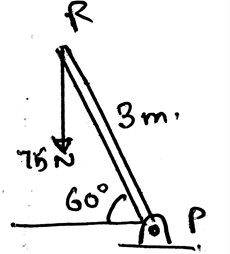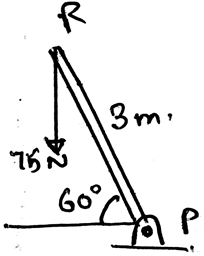Courses

# Test: Frictional Forces On Screws

## 15 Questions MCQ Test Engineering Mechanics | Test: Frictional Forces On Screws

Description
This mock test of Test: Frictional Forces On Screws for Mechanical Engineering helps you for every Mechanical Engineering entrance exam. This contains 15 Multiple Choice Questions for Mechanical Engineering Test: Frictional Forces On Screws (mcq) to study with solutions a complete question bank. The solved questions answers in this Test: Frictional Forces On Screws quiz give you a good mix of easy questions and tough questions. Mechanical Engineering students definitely take this Test: Frictional Forces On Screws exercise for a better result in the exam. You can find other Test: Frictional Forces On Screws extra questions, long questions & short questions for Mechanical Engineering on EduRev as well by searching above.
QUESTION: 1

### In general cases the screws are used as __________

Solution:

The screws are generally used as the fasteners. The main thing about the screws is that the friction helps the holding of the structures. Also screws help make the structure join fast and efficiently. Thus the name fasteners.

QUESTION: 2

### Which type of the screws are used in the machines?

Solution:

The square threaded screws are used in the machines. As the screws are generally used as the fasteners. The main thing about the screws is that the friction helps the holding of the structures. Also screws help make the structure join fast and efficiently

QUESTION: 3

### Why are square threaded screws are used in the machines?

Solution:

The square threaded screws are used in the machines. As these screws are generally used as the fasteners. The main thing about these screws is that the friction helps the holding of the structures. Also screws help make the structure join fast and efficiently. And in these screws forces act along the axis of screws.

QUESTION: 4

The angle of the threading in the screw is determined by which of the following trigonometric function?

Solution:

The angle of the threading in the screw is determined by inverse tangent trigonometric function. This is the generalised form of the function which is being used so as to get the value of the angle. In wedges the same function is used so as to find the angle of wedge.

QUESTION: 5

In the determination of the angle of the screw, the ratio kept in the inverse tangent function is the ratio of ________

Solution:

The angle of the threading in the screw is determined by inverse tangent trigonometric function. This is the generalised form of the function which is being used so as to get the value of the angle. In wedges the same function is used so as to find the angle of wedge. And in both the case the ratio is the vertical is to horizontal.

QUESTION: 6

The horizontal distance used in the inverse trigonometry function is called as _____________

Solution:

The angle of the threading in the screw is determined by inverse tangent trigonometric function. This is the generalised form of the function which is being used so as to get the value of the angle. In wedges the same function is used so as to find the angle of wedge. And thus the horizontal distance is called as lead.

QUESTION: 7

In screws there is application of two and three force members. The basic difference between the two and the three force members used is ___________

Solution:

The definition of the two force member only defines that the forces are being acted on the two points on the body. So does is the definition of the three forces members. The points of action of the three forces are three.

QUESTION: 8

Determine the moment of the force along point P (There is screw present at P, about which the rotation is there).Solution:

As we know that the moment is the cross product of the distance and the force we will try to apply the same here. We see that the perpendicular distance is 1.5 (3cos60). Thus we get the distance. And hence multiply it with the force, 75×1.5. Because the force component perpendicular to the distance need to be taken.

QUESTION: 9

When we apply the equilibrium equations in the free body diagram of the screw, for two force members to be in equilibrium then _________

Solution:

Simply, for the equilibrium the net force must be zero. That is the forces must be collinear if equilibrium is to be established. And they must be in the opposite directions. They also must be of same magnitude, cancelling each other.

QUESTION: 10

Which of the following statement for the three force system applied in the screws is true?

Solution:

The forces in the three force system are not always in the equilibrium. The equilibrium is established when the forces cancels out each other. Also when the net moment is zero. Then only the equilibrium is established in the three force system.

QUESTION: 11

Which of the following is correct for a screw being rotated?

Solution:

The couple moment depends only on the distance vector between the forces. The radius vector of the forces are not making the couple depended over it. But the distance vector is driven by subtracting the radius vectors of the forces. This means that, moment of the couple doesn’t directly depend on the radius vector of forces.

QUESTION: 12

If the forces acting on the couple acting on the screw are in the same direction, that is they are not in the opposite direction as always they are, then?

Solution:

The forces are always in the opposite direction for the couple moment. But if they don’t act like the same that is they change their directions and align themselves in the same direction then the couple is not possible.

QUESTION: 13

For equilibrium the net force acting on the screw is zero.

Solution:

The equilibrium is only attained if the net force on the body tends to be equal to zero. Thus the forces cancel out. If this happens there is no motion of the body along any direction and hence the body is said to be in equilibrium. The body here is a rigid body.

QUESTION: 14

In the screw collinear forces act, what is a collinear system of forces?

Solution:

The force system having all the forces emerging from a point is called the collinear system of force. This is a type of system of the force, which is easy in the simplification. This is because as the forces are the vector quantity, the vector math is applied and the simplification is done.

QUESTION: 15

Determine the smallest force applied at R which creates the same moment about P as by 75N. (There is presence of screw at P, about which the rotation is being there)Solution:

As we know that the moment is the cross product of the distance and the force we will try to apply the same here. We see that the perpendicular distance is 3m. Thus we get the distance. And hence multiply it with the force, the moment = 112.5Nm. Because the force component perpendicular to the distance need to be taken.